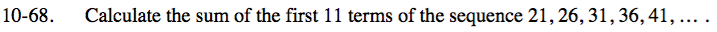### Home > INT3 > Chapter Ch10 > Lesson 10.1.5 > Problem10-68

10-68.

Calculate the sum of the first 11 terms of the sequence 21, 26, 31, 36, 41, … . Homework Help ✎t(n) = 5n + 16

${ S_{11}=\frac{n}{2}(t(1)+t(n))=\frac{11}{2}(21 + 71)}$

S11 = 506